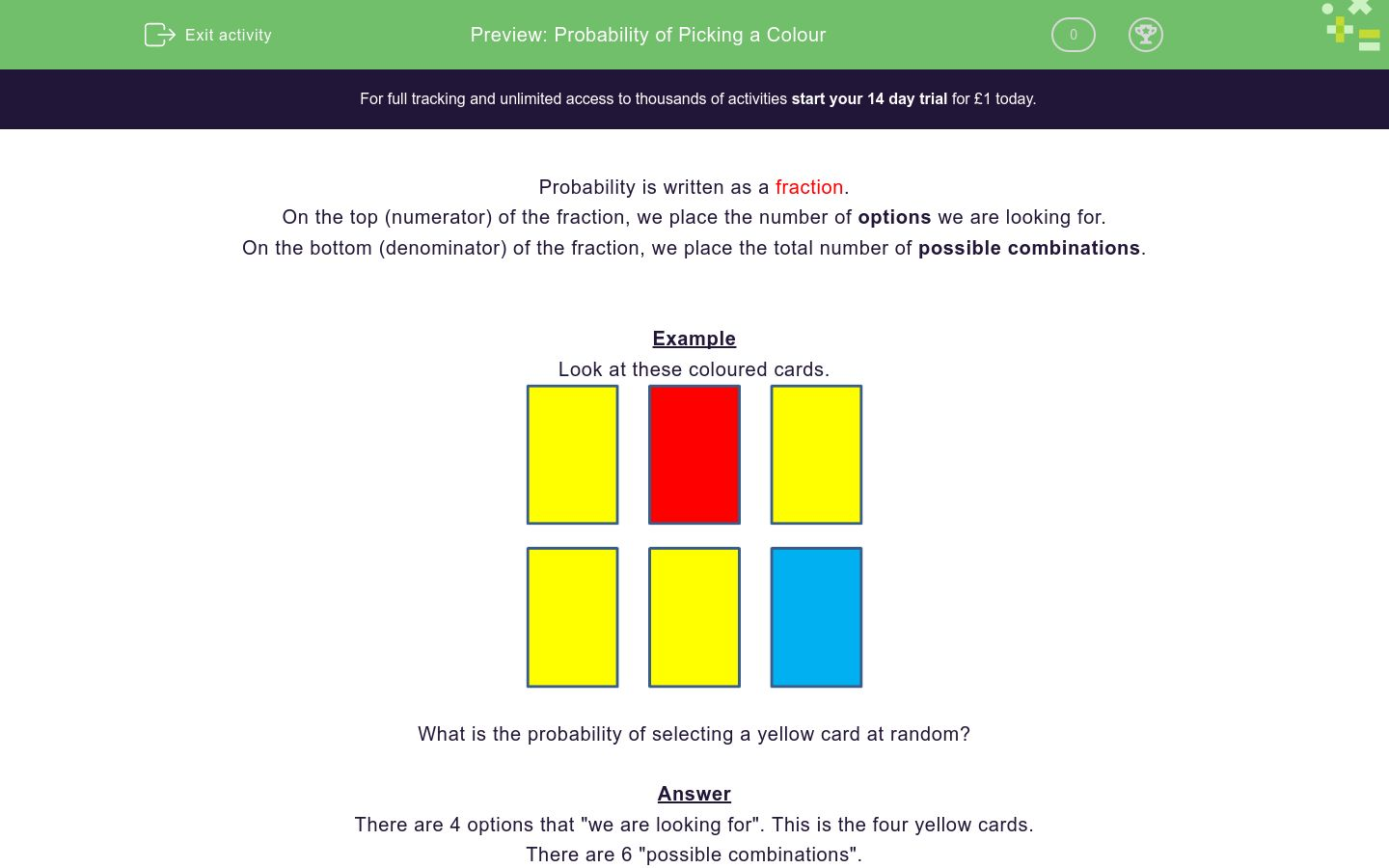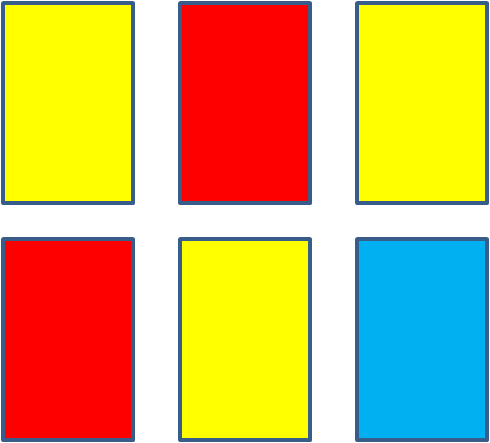# Probability of Picking a Colour

In this worksheet, students calculate the probability of choosing a certain colour.Key stage:  KS 3

Curriculum topic:   Probability

Curriculum subtopic:   Understand Probability Outcomes

Difficulty level:### QUESTION 1 of 10

Probability is written as a fraction.

On the top (numerator) of the fraction, we place the number of options we are looking for.

On the bottom (denominator) of the fraction, we place the total number of possible combinations.

Example

Look at these coloured cards.What is the probability of selecting a yellow card at random?

There are 4 options that "we are looking for". This is the four yellow cards.

There are 6 "possible combinations".

The probability of choosing a yellow card is 4/6 which reduces to 2/3.

Look at these coloured cards.What is the probability of selecting a red card at random?

(write the answer as a fraction in its lowest terms using the / sign e.g. 3/4)

Look at these coloured cards.What is the probability of selecting a blue card at random?

(write the answer as a fraction in its lowest terms using the / sign e.g. 3/4)

Look at these coloured cards.What is the probability of selecting a yellow card at random?

(write the answer as a fraction in its lowest terms using the / sign e.g. 3/4)

Look at these coloured cards.What is the probability of selecting a red card at random?

(write the answer as a fraction in its lowest terms using the / sign e.g. 3/4)

Look at these coloured cards.What is the probability of selecting a green card at random?

(write the answer as a fraction in its lowest terms using the / sign e.g. 3/4)

Look at these coloured cards.What is the probability of selecting a red card at random?

(write the answer as a fraction in its lowest terms using the / sign e.g. 3/4)

Look at these coloured cards.What is the probability of selecting a yellow card at random?

(write the answer as a fraction in its lowest terms using the / sign e.g. 3/4)

Look at these coloured cards.What is the probability of selecting a blue card at random?

(write the answer as a fraction in its lowest terms using the / sign e.g. 3/4)

Look at these coloured cards.What is the probability of selecting a blue card at random?

(write the answer as a fraction in its lowest terms using the / sign e.g. 3/4)

Look at these coloured cards.What is the probability of selecting a red card at random?

(write the answer as a fraction in its lowest terms using the / sign e.g. 3/4)

• Question 1

Look at these coloured cards.What is the probability of selecting a red card at random?

(write the answer as a fraction in its lowest terms using the / sign e.g. 3/4)

1/6
• Question 2

Look at these coloured cards.What is the probability of selecting a blue card at random?

(write the answer as a fraction in its lowest terms using the / sign e.g. 3/4)

1/6
• Question 3

Look at these coloured cards.What is the probability of selecting a yellow card at random?

(write the answer as a fraction in its lowest terms using the / sign e.g. 3/4)

1/2
• Question 4

Look at these coloured cards.What is the probability of selecting a red card at random?

(write the answer as a fraction in its lowest terms using the / sign e.g. 3/4)

1/3
• Question 5

Look at these coloured cards.What is the probability of selecting a green card at random?

(write the answer as a fraction in its lowest terms using the / sign e.g. 3/4)

0
EDDIE SAYS
0/6 = 0
• Question 6

Look at these coloured cards.What is the probability of selecting a red card at random?

(write the answer as a fraction in its lowest terms using the / sign e.g. 3/4)

3/8
• Question 7

Look at these coloured cards.What is the probability of selecting a yellow card at random?

(write the answer as a fraction in its lowest terms using the / sign e.g. 3/4)

3/8
• Question 8

Look at these coloured cards.What is the probability of selecting a blue card at random?

(write the answer as a fraction in its lowest terms using the / sign e.g. 3/4)

1/4
• Question 9

Look at these coloured cards.What is the probability of selecting a blue card at random?

(write the answer as a fraction in its lowest terms using the / sign e.g. 3/4)

1/2
• Question 10

Look at these coloured cards.What is the probability of selecting a red card at random?

(write the answer as a fraction in its lowest terms using the / sign e.g. 3/4)

1/3
---- OR ----

Sign up for a £1 trial so you can track and measure your child's progress on this activity.

### What is EdPlace?

We're your National Curriculum aligned online education content provider helping each child succeed in English, maths and science from year 1 to GCSE. With an EdPlace account you’ll be able to track and measure progress, helping each child achieve their best. We build confidence and attainment by personalising each child’s learning at a level that suits them.

Get started